# Forecasting Process Details

### Smoothing Model Calculations

Subsections:

The descriptions and properties of various smoothing methods can be found in Gardner (1985); Chatfield (1978); Bowerman and O’Connell (1979). The following section summarizes the smoothing model computations.

Given a time series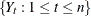, the underlying model assumed by the smoothing models has the following (additive seasonal) form: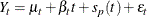where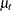represents the time-varying mean term.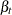represents the time-varying slope.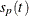represents the time-varying seasonal contribution for one of the
seasons.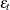are disturbances.

For smoothing models without trend terms,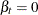; and for smoothing models without seasonal terms,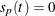. Each smoothing model is described in the following sections.

At each time, the smoothing models estimate the time-varying components described above with the smoothing state. After initialization, the smoothing state is updated for each observation using the smoothing equations. The smoothing state at the last nonmissing observation is used for predictions.

#### Smoothing State and Smoothing Equations

Depending on the smoothing model, the smoothing state at timeconsists of the following: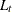is a smoothed level that estimates.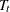is a smoothed trend that estimates.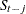,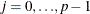, are seasonal factors that estimate.

The smoothing process starts with an initial estimate of the smoothing state, which is subsequently updated for each observation by using the smoothing equations.

The smoothing equations determine how the smoothing state changes as time progresses. Knowledge of the smoothing state at time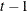and that of the time series value at timeuniquely determine the smoothing state at time. The smoothing weights determine the contribution of the previous smoothing state to the current smoothing state. The smoothing equations for each smoothing model are listed in the following sections.

#### Smoothing State Initialization

Given a time series, the smoothing process first computes the smoothing state for time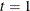. However, this computation requires an initial estimate of the smoothing state at time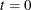, even though no data exists at or before time.

An appropriate choice for the initial smoothing state is made by backcasting from time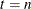toto obtain a prediction at. The initialization for the backcast is obtained by regression with constant and linear terms and seasonal dummies (additive or multiplicative) as appropriate for the smoothing model. For models with linear or seasonal terms, the estimates obtained by the regression are used for initial smoothed trend and seasonal factors; however, the initial smoothed level for backcasting is always set to the last observation,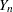.

The smoothing state at timeobtained from the backcast is used to initialize the smoothing process from timeto(Chatfield and Yar, 1988).

For models with seasonal terms, the smoothing state is normalized so that the seasonal factorsforsum to zero for models that assume additive seasonality and average to one for models (such as Winters method) that assume multiplicative seasonality.